Test: Operational Amplifiers

# Test: Operational Amplifiers

Test Description

## 20 Questions MCQ Test GATE Electrical Engineering (EE) 2023 Mock Test Series | Test: Operational Amplifiers

Test: Operational Amplifiers for Electrical Engineering (EE) 2023 is part of GATE Electrical Engineering (EE) 2023 Mock Test Series preparation. The Test: Operational Amplifiers questions and answers have been prepared according to the Electrical Engineering (EE) exam syllabus.The Test: Operational Amplifiers MCQs are made for Electrical Engineering (EE) 2023 Exam. Find important definitions, questions, notes, meanings, examples, exercises, MCQs and online tests for Test: Operational Amplifiers below.
Solutions of Test: Operational Amplifiers questions in English are available as part of our GATE Electrical Engineering (EE) 2023 Mock Test Series for Electrical Engineering (EE) & Test: Operational Amplifiers solutions in Hindi for GATE Electrical Engineering (EE) 2023 Mock Test Series course. Download more important topics, notes, lectures and mock test series for Electrical Engineering (EE) Exam by signing up for free. Attempt Test: Operational Amplifiers | 20 questions in 60 minutes | Mock test for Electrical Engineering (EE) preparation | Free important questions MCQ to study GATE Electrical Engineering (EE) 2023 Mock Test Series for Electrical Engineering (EE) Exam | Download free PDF with solutions
 1 Crore+ students have signed up on EduRev. Have you?
Test: Operational Amplifiers - Question 1

### For the circuit shown in fig. the input resistance is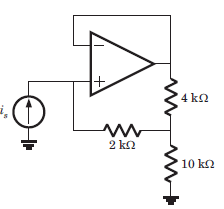Detailed Solution for Test: Operational Amplifiers - Question 1

Since op-amp is ideal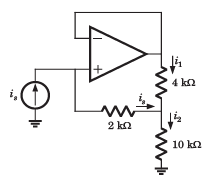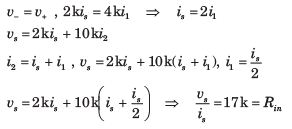Test: Operational Amplifiers - Question 2

### In the circuit of fig. the op-amp slew rate is SR = 0.5 V/μs. If the amplitude of input signal is 0.02 V, then the maximum frequency that may be used is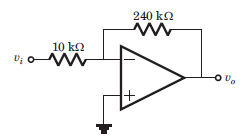Detailed Solution for Test: Operational Amplifiers - Question 2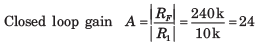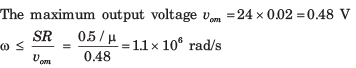Test: Operational Amplifiers - Question 3

### In the circuit of fig. the input offset voltage and input offset current are Vio = 4 mV and Iio = 150 nA. The total output offset voltage is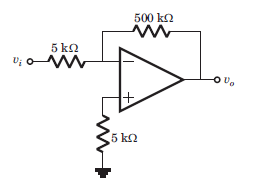Detailed Solution for Test: Operational Amplifiers - Question 3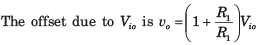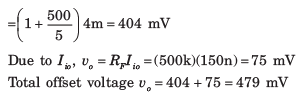Test: Operational Amplifiers - Question 4

io = ?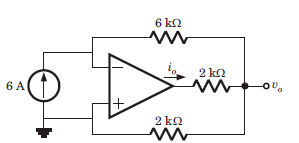Detailed Solution for Test: Operational Amplifiers - Question 4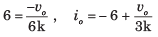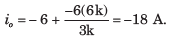Test: Operational Amplifiers - Question 5

If ‘V’ is the voltage phasor and ‘I’ is the current phasor, then VI represents

Detailed Solution for Test: Operational Amplifiers - Question 5

Apparent Power (S): It is defined as the product of r.m.s value of voltage (V) and current (1). It is denoted by S. S = V/I Volt Ampere

Test: Operational Amplifiers - Question 6

Consider the circuit shown below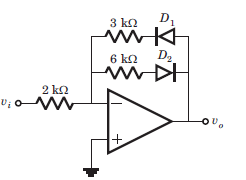Que: If vi = -2 V, then output vo is

Detailed Solution for Test: Operational Amplifiers - Question 6

If vi < 0, then v0 > 0, D1 blocks and D2 conducts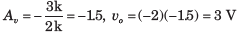Test: Operational Amplifiers - Question 7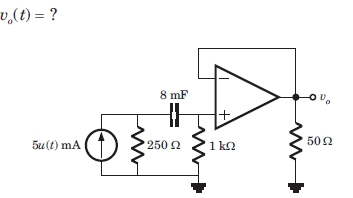Detailed Solution for Test: Operational Amplifiers - Question 7

Voltage follower v0 = v = v+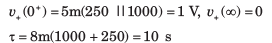Test: Operational Amplifiers - Question 8

The circuit shown in fig. is at steady state before the switch opens at t = 0. The vc(t) for t > 0 is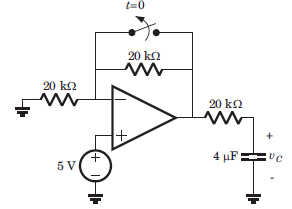Detailed Solution for Test: Operational Amplifiers - Question 8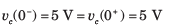For t > 0 the equivalent circuit is shown in fig.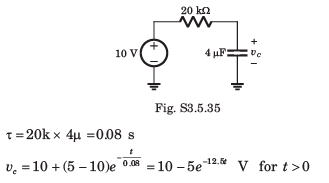Test: Operational Amplifiers - Question 9

The LED in the circuit of fig. will be on if vis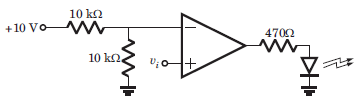Detailed Solution for Test: Operational Amplifiers - Question 9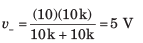When v+ > 5 V, output will be positive and LED will be on. Hence (C) is correct.

Test: Operational Amplifiers - Question 10

In the circuit of fig. the CMRR of the op-amp is 60 dB. The magnitude of the vo is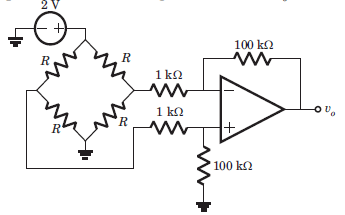Detailed Solution for Test: Operational Amplifiers - Question 10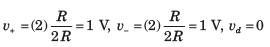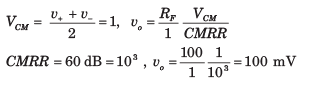Test: Operational Amplifiers - Question 11

The analog multiplier X of fig. has the characteristics vp = v1v2 The output of this circuit is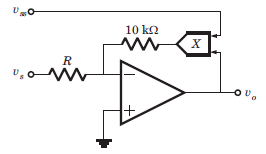Detailed Solution for Test: Operational Amplifiers - Question 11

v+ = 0 = v-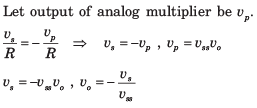Test: Operational Amplifiers - Question 12

If the input to the ideal comparator shown in fig. is a sinusoidal signal of 8 V (peak to peak) without any DC component, then the output of the comparator has a duty cycle of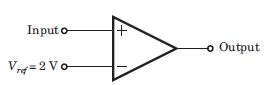Detailed Solution for Test: Operational Amplifiers - Question 12

When vi > 2 V, output is positive. When vi < 2 V, output is negative.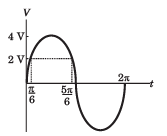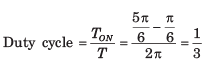Test: Operational Amplifiers - Question 13

In the op-amp circuit given in fig. the load current iL is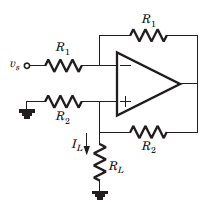Detailed Solution for Test: Operational Amplifiers - Question 13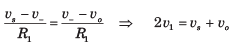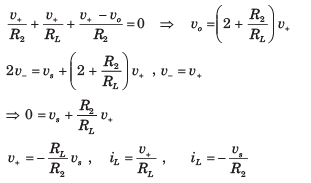Test: Operational Amplifiers - Question 14

In the circuit of fig. output voltage is |vo| =1 V for a certain set of ω, R, an C. The |vo| will be 2 V if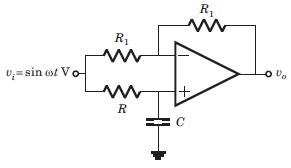Detailed Solution for Test: Operational Amplifiers - Question 14

This is a all pass circuit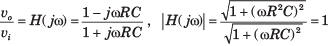Thus when ω and R is changed, the transfer function is unchanged

Test: Operational Amplifiers - Question 15

In the circuit of fig. the 3 dB cutoff frequency is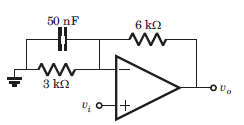Detailed Solution for Test: Operational Amplifiers - Question 15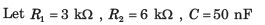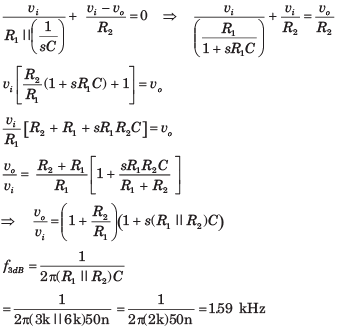Test: Operational Amplifiers - Question 16

The phase shift oscillator of fig. operate at f = 80 kHz. The value of resistance RF is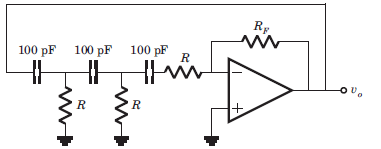Detailed Solution for Test: Operational Amplifiers - Question 16

The oscillation frequency is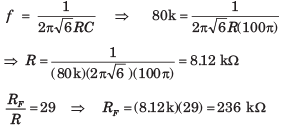Test: Operational Amplifiers - Question 17

The value of C required for sinusoidal oscillation of frequency 1 kHz in the circuit of fig. is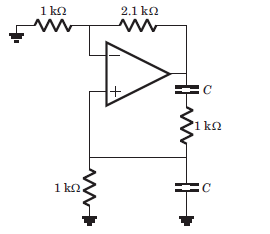Detailed Solution for Test: Operational Amplifiers - Question 17

This is Wien-bridge oscillator. The ratio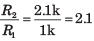is greater than 2. So there will be
oscillation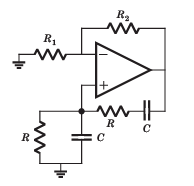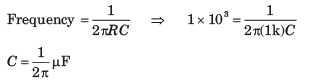Test: Operational Amplifiers - Question 18

In the circuit shown in fig. the op-amp is ideal. If βF = 60, then the total current supplied by the 15 V source is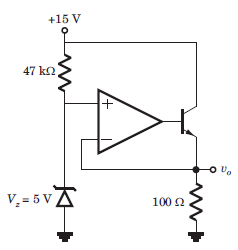Detailed Solution for Test: Operational Amplifiers - Question 18

v+ = 5V = v = VE ,

The input current to the op-amp is zero.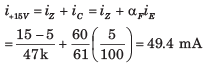Test: Operational Amplifiers - Question 19

In the circuit in fig. both transistor Q1 and Q2 are identical. The output voltage at T = 300 K is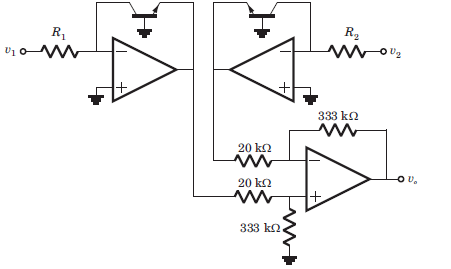Detailed Solution for Test: Operational Amplifiers - Question 19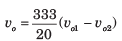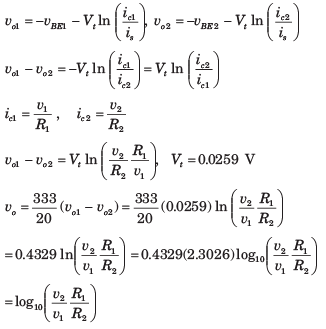Test: Operational Amplifiers - Question 20

In the op-amp series regulator circuit of fig. Vz = 62. V, VBE = 0.7 V and β = 60. The output voltage vo is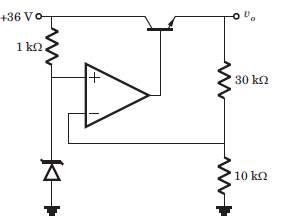Detailed Solution for Test: Operational Amplifiers - Question 20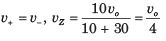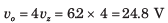## GATE Electrical Engineering (EE) 2023 Mock Test Series

22 docs|274 tests
 Use Code STAYHOME200 and get INR 200 additional OFF Use Coupon Code
Information about Test: Operational Amplifiers Page
In this test you can find the Exam questions for Test: Operational Amplifiers solved & explained in the simplest way possible. Besides giving Questions and answers for Test: Operational Amplifiers, EduRev gives you an ample number of Online tests for practice

## GATE Electrical Engineering (EE) 2023 Mock Test Series

22 docs|274 tests